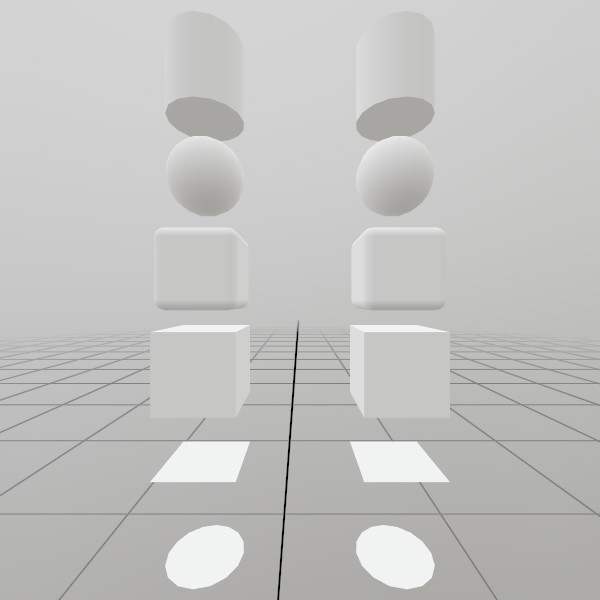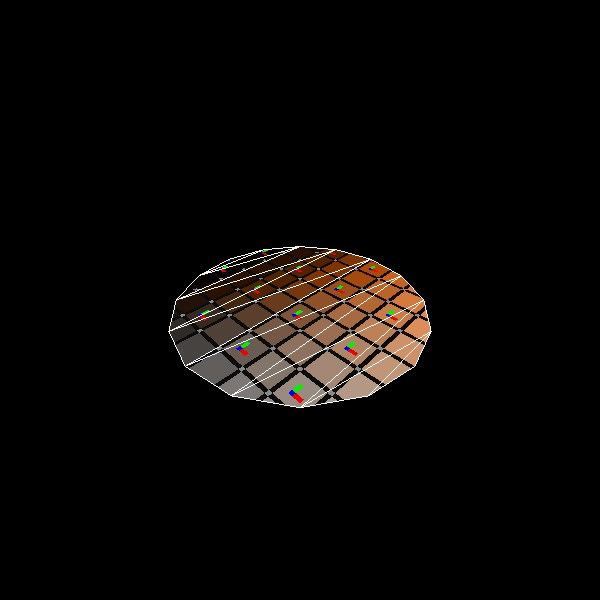# Mesh.GenerateCircle

``````static Mesh GenerateCircle(float diameter, int spokes, bool doubleSided)
``````

Generates a circle on the XZ axis facing up that is pre-sized to the given diameter. UV coordinates correspond to a unit circle centered at 0.5, 0.5! That is, the right-most point on the circle has UV coordinates 1, 0.5 and the top-most point has UV coordinates 0.5, 1.

NOTE: This generates a completely new Mesh asset on the GPU, and is best done during ‘initialization’ of your app/scene.

float diameter The diameter of the circle in meters, or 2*radius. This is the full length from one side to the other.
int spokes How many vertices compose the circumference of the circle? Clamps to a minimum of 3. More is smoother, but less performant.
bool doubleSided Should both sides of the circle be rendered?
RETURNS: Mesh A circle mesh, pre-sized to the given dimensions.
``````static Mesh GenerateCircle(float diameter, Vec3 planeNormal, Vec3 planeTopDirection, int spokes, bool doubleSided)
``````

Generates a circle with an arbitrary orientation that is pre-sized to the given diameter. UV coordinates start at the top left indicated with ‘planeTopDirection’ and correspond to a unit circle centered at 0.5, 0.5.

NOTE: This generates a completely new Mesh asset on the GPU, and is best done during ‘initialization’ of your app/scene.

float diameter The diameter of the circle in meters, or 2*radius. This is the full length from one side to the other.
Vec3 planeNormal What is the normal of the surface this circle is generated on?
Vec3 planeTopDirection A normal defines the plane, but this is technically a rectangle on the plane. So which direction is up? It’s important for UVs, but doesn’t need to be exact. This function takes the planeNormal as law, and uses this vector to find the right and up vectors via cross-products.
int spokes How many vertices compose the circumference of the circle? Clamps to a minimum of 3. More is smoother, but less performant.
bool doubleSided Should both sides of the circle be rendered?
RETURNS: Mesh A circle mesh, pre-sized to the given dimensions.

## Examples

### Generating a Mesh and ModelHere’s a quick example of generating a mesh! You can store it in just a Mesh, or you can attach it to a Model for easier rendering later on.

``````// Do this in your initialization
Mesh  circleMesh  = Mesh.GenerateCircle(0.4f);
Model circleModel = Model.FromMesh(circleMesh, Default.Material);
``````

Drawing both a Mesh and a Model generated this way is reasonably simple, here’s a short example! For the Mesh, you’ll need to create your own material, we just loaded up the default Material here.

``````Matrix circleTransform = Matrix.T(0, -1.5f, 0);
circleMesh.Draw(Default.Material, circleTransform);

circleTransform = Matrix.T(1, -1.5f, 0);
circleModel.Draw(circleTransform);
``````

### UV and Face layout

Here’s a test image that illustrates how this mesh’s geometry is laid out.``````meshCircle = Mesh.GenerateCircle(1);
``````

Found an issue with these docs, or have some additional questions? Create an Issue on Github!